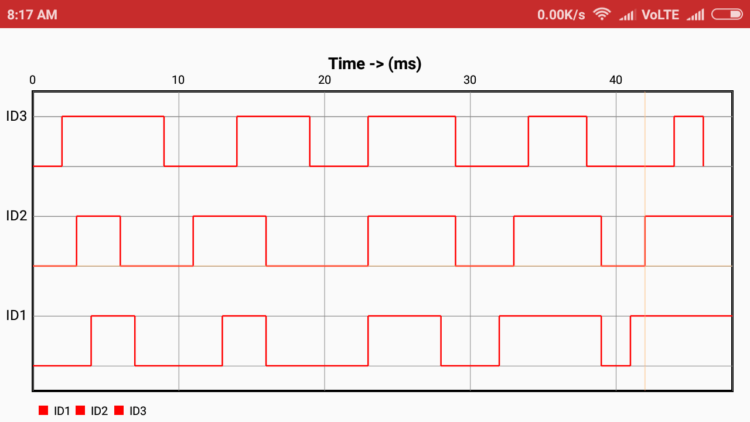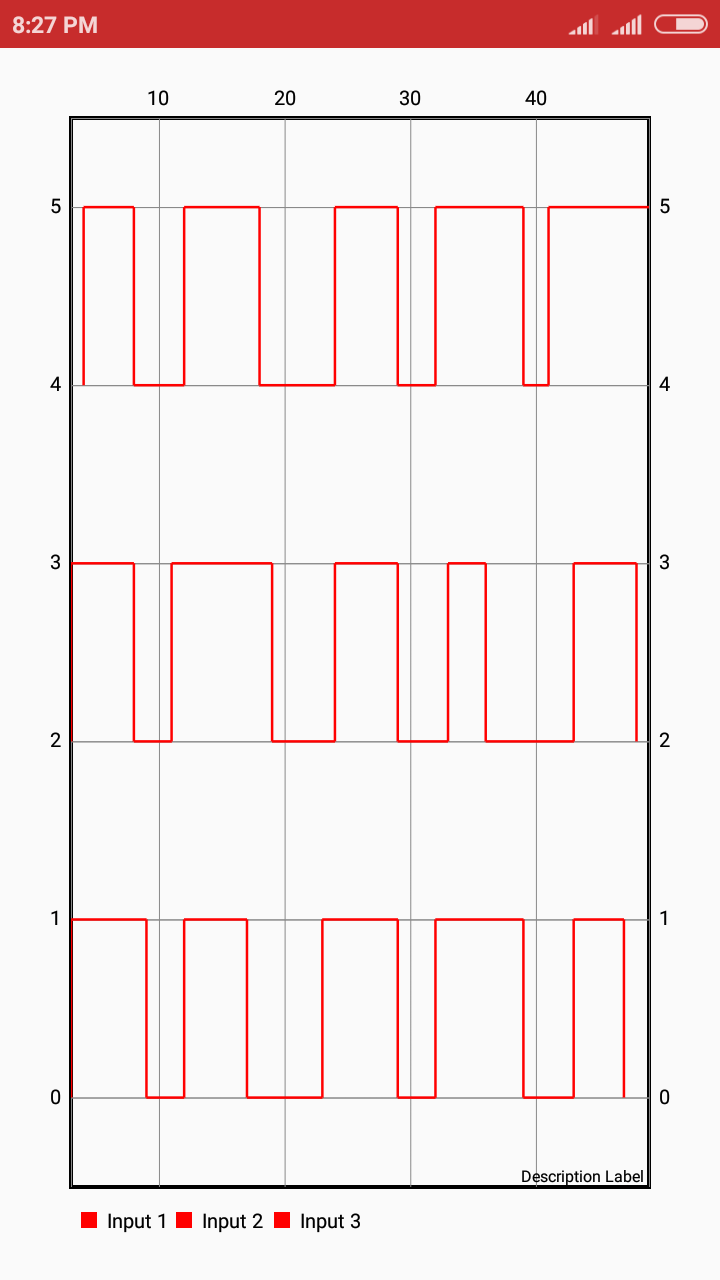## Plotting Digital Logic Lines In PSLab Android App

The PSLab device offers the Logic Analyzer functionality. A Logic Analyzer is a laboratory instrument that can capture and display digital signals from a digital system or circuit. It is similar to what an oscilloscope is for analog signals and is used to study timing relationship between different logic lines. It plots the logic lines/timing diagram which tells us the information about the state of the Digital System at any instant of time. For example, in the image below we can study the states of digital signals from channels ID1, ID2, ID3 at different times and find parameters like the propagation delay. It’s also used to find errors in Integrated Circuits (ICs) and debug logic circuits.How I plotted ideal logic lines using MPAndroid Chart library?

Conventional method of adding data points results in the plot as illustrated in the image below. By conventional method I mean basically adding Y-axis (logic state) values corresponding to X-axis values (timestamp).

In the above plot, logic lines follow non-ideal behaviour i.e they take some time in changing their state from high to low. This non-ideal behaviour of these lines increases when the user zooms in graph to analyse timestamps.

Solution to how we can achieve ideal behaviour of logic lines:

A better solution is to make use of timestamps for generating logic lines i.e time instants at which logic made a transition from HIGH -> LOW or LOW -> HIGH. Lets try to figure out with an example:

Timestamps = { 1, 3, 5, 8, 12 } and initial state is HIGH ( i.e at t = 0, it’s HIGH ). This implies that at t = 1, transition from HIGH to LOW took place so at t = 0, it’s HIGH, t = 1 it’s both HIGH and LOW,  at t = 2 it’s LOW.
Now at t = 0 & t = 2, you can simple put y = 1 and 0 respectively. But how do you add data-point for t = 1. Trick is to see how transition is taking place, if it’s HIGH to LOW then add first 1 for t = 1 and then 0 for t = 1.
So the set of points look something like this:

( Y, X ) ( LOGIC , TIME ) -> ( 1, 0 ) ( 1, 1 ) ( 0, 1) ( 0, 2 ) ( 0, 3 ) ( 1, 3 )  ( 1, 4 ) …

Code snippet for adding coordinates in this fashion:

```int[] time = timeStamps.get(j);
for (int i = 0; i < time.length; i++) {
if (initialState) {
// Transition from HIGH -> LOW
} else {
// Transition from LOW -> HIGH